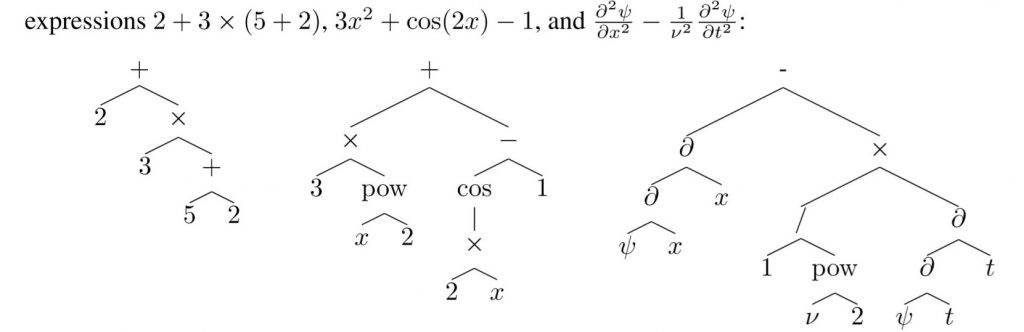In December, Lample and Charton from Facebook’s Artificial Intelligence Research group published a paper stating that they had created an AI application that outperformed systems such as Matlab and Mathematica when presented with complex equations. Is this a huge leap forward or just an obvious extension of maths solving systems that have been around for years? Let’s take a look.

Mathematics is not something that we learn by trial and error. There’s a great joke for machine learning hiring that shows the ridiculousness of this for simple addition, as well as the long standing over engineering of using machine learning for what I hope is a defunct technical interview exercise of fizz-buzz.

We start with addition and subtraction as very visible activities1 and we build on this with multiplication and division as combinations of addition or subtraction. We learn our times tables by rote and, with a little practise, we can extrapolate complex multiplications and factors based on these2. We then learn formulae for specific situations, and new symbols that do things differently, and again we memorised what they do and apply this with whatever numbers we are given.

When we get to differentiation and integration, we are given a further set of results and rules to memorise. It can become more tricky to determine which rule to apply with complex equations, and this is achieved with practise, which boils down to spotting how to split the equation correctly.

We have prior knowledge and a series of rules to apply. This is prime for procedural programming. It’s how calculators work. It’s how Mathematica and MATLAB work. It’s why students who are stuck on assignments can type equations into Google and get the correct answer3.

However, in order to deal with the practically infinite variation in equations that can be put into these applications, the rules that are hard coded to provide solutions are inefficient for all but the most straight forward4 problems without needing the human operator to simplify the input.

The best human mathematicians can spot the patterns in the equations in the same way that musicians hear the individual instruments in an orchestra. In this way you can get to an answer far more efficiently than by following a long process. The work by Lample and Charton aims to replicate the pattern recognition that humans use innately. Much of the machine learning work in natural language processing (NLP) splits sentences into sequences and is then looking for patterns in those sequences. They realised that solving equations takes the same approach.From Lample and Charton 2019: Three examples of splitting equations onto logical blocks for precedence. These are very similar to linguistic trees.

While there was a one to one mapping between equations and trees, multiple trees can lead to the same answer. Much of their paper details how they generated the data sets for their experiments, which is fascinating in itself, and if that interests you then please read the original paper, but the key point being that training sets were 20 or 40 million examples. Using seq2seq they built a model to predict the solution given an input. This was key – recognising that maths is no more than turning one sentence into another. As such, they could skip the procedural solving methods and simply5 “translate” the equations to their answers.

I’m a huge fan of cross domain research – some of the biggest leaps in science have come from someone applying techniques from different areas. In this instance, it appears that language techniques have a huge impact on solving maths problems. In testing against Mathematica, MATLAB and Maple, the seq2seq model significantly outperformed them, both in terms of speed and accuracy. While the 500 equations is a decent sample size, I’m sure that it would be possible to find a further 500 on which the commercial solvers would outperform the Lample and Charton model, but as the authors themselves say at the end of their paper, integrating this type of solution into those solvers alongside procedural solutions is definitely worthwhile.

1. Fingers, toys, chocolates, etc 🙂
2. The more you do this the more it stays easily accessible in your brain, but how often do you really need to recall the factors of 12464 on a day to day basis?
3. Assuming you know how to convert mathematical typesetting from your problem sheet into computational typesetting…
4. I do use this term as a relative as you can solve very complex equations with these applications, but only up to a point before you will start getting errors.
5. I’m not meaning to make this sound easy :).
Dr Janet is a Molecular Biochemistry graduate from Oxford University with a doctorate in Computational Neuroscience from Sussex. I’m currently studying for a third degree in Mathematics with Open University.

During the day, and sometimes out of hours, I work as a Chief Science Officer. You can read all about that on my LinkedIn page.